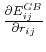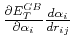Next: Configuration Parameters Up: Generalized Born Implicit Solvent Previous: Theoretical Background   Contents   Index

## 3-Phase Calculation

The GBIS algorithm requires three phases of calculation, with each phase containing an iteration over all atom pairs with the cutoff. In phase 1, the screening of atom pairs is summed; at the conclusion of phase 1, the Born radii are calculated. In phase 2, theforce contribution (hereafter called the dEdr force) is calculated as well as the partial derivative of the Born radii with respect to the atom positions,. In phase 3, theforce contribution (hereafter called the dEda force) is calculated.

http://www.ks.uiuc.edu/Research/namd/